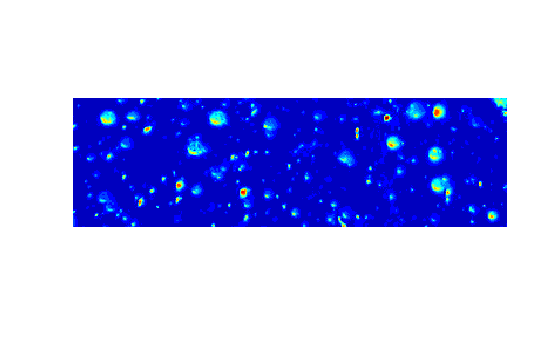# grayslice

Convert grayscale image to indexed image using multilevel thresholding

## Syntax

``X = grayslice(I,N)``
``X = grayslice(I,thresholds)``

## Description

example

````X = grayslice(I,N)` converts a grayscale image to an indexed image by using multilevel thresholding approach. The function automatically calculates the threshold values based on `N`. To learn more about threshold calculation, see Algorithms.```

example

````X = grayslice(I,thresholds)` returns an indexed image by multilevel thresholding of input image using a specified set of thresholds.```

## Examples

collapse all

Read grayscale image into the workspace.

`I = imread('snowflakes.png');`

Threshold the intensity image, returning an indexed image.

`X = grayslice(I,16);`

Display the original image and the indexed image, using one of the standard colormaps.

`imshow(I)````figure imshow(X,jet(16))```Read a grayscale image into the workspace. Display the image.

```I = imread('coins.png'); imshow(I)```Specify the threshold values for multilevel thresholding.

`thresholds = [45 65 84 108 134 157 174 189 206 228];`

Convert the input grayscale image to an indexed image.

`X = grayslice(I,thresholds);`

Display the indexed image. Set the colormap of the indexed image to `jet`. The length of colormap, `m`, is the maximum intensity value in the indexed image.

```m = double(max(X(:))); figure imshow(X,colormap(jet(m)))```## Input Arguments

collapse all

Input grayscale image, specified as a m-by-n numeric matrix.

Data Types: `single` | `double` | `int16` | `uint8` | `uint16`

Number of threshold values, specified as a positive scalar. The value represents the total number of thresholds to be used for multilevel thresholding.

Data Types: `single` | `double` | `int16` | `uint8` | `uint16`

Set of thresholds, specified as a numeric vector. The number of threshold values to be used for multilevel thresholding is equal to `length(thresholds)`.

Image Data TypeRange of Valid Threshold Values
`uint8`[0, 255]
`int16` or `uint16`[0, 65535]
`single` or `double`[0, 1]

Note

Before thresholding an image of data type `int16`, the `grayslice` function converts the image to `uint16` by adding 32,768 to each pixel. Consider this additive offset when specifying thresholds for input images of data type `int16`.

Data Types: `single` | `double` | `int16` | `uint8` | `uint16`

## Output Arguments

collapse all

Output indexed image, returned as a m-by-n matrix of the same size as the input grayscale image. The data type of `X` depends on the number of threshold values used for multilevel thresholding.

• If the number of threshold values is less than 256, then `X` is of data type `uint8`. In this case, the range of intensity values in `X` is either [0, `N`-1] or [0, `length`(`thresholds`)].

• If the number of threshold values is greater than or equal to 256, then `X` is of data type `double`. In this case, the range of intensity values in `X` is either [1, `N`] or [1, `length`(`thresholds`)+1].

Data Types: `uint8` | `double`

## Tips

• You can view the thresholded image using `imshow(X,map)` with a colormap of appropriate length.

## Algorithms

The function performs multilevel thresholding of the input grayscale image and returns an indexed image as the output. If you specify the number of thresholds `N`, then `grayslice` assigns pixels to `N` indices according to the these thresholds.

• The first index in `X` consists of the grayscale pixels in the range $max_intensity×\left[0,\text{\hspace{0.17em}}\frac{1}{N}\right)$

• The k-th index in `X` consists of the grayscale pixels in the range $max_intensity×\left[\frac{k-1}{N},\text{\hspace{0.17em}}\frac{k}{N}\right)$

• The last index in `X` consists of the grayscale pixels in the range $max_intensity×\left[\frac{N-1}{N},\text{\hspace{0.17em}}1\right]$.

max_intensity depends on the data type of the input image.

Image Data Typemax_intensity
`uint8`255
`int16` or `uint16`65535
`single` or `double`1

Note

Before thresholding an image of data type `int16`, the `grayslice` function converts the image to `uint16` by adding 32,768 to each pixel.

## Version History

Introduced before R2006a

expand all